Derivation of wave equation

## Linear Methods of Applied Mathematics Evans M. Harrell II and James V. Herod*

version of 8 August 1996

The physics and mathematics of the vibrating string were studied by Jean le Rond d'Alembert, and later by Joseph Louis Lagrange, Leonhard Euler, and Daniel Bernoulli, who gave a satisfactory discussion of the physics of the vibrating string. I have not been able to locate a detailed discussion of Bernoulli's derivation of the wave equation, but it is likely that he based it on an energy principle, somewhat as follows. First let us recall that in classical mechanics, Newton's equations for the motion of a particle are equivalent to the vanishing of the first variation of the Lagrangian action integral,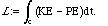The kinetic energy is usually of the form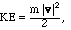while the potential energy PE may be a function of position, U(q). (I call the position q to avoid confusion with an x used below). The action integral is calculated along a trajectory C = (q(t)) beginning at position and time coordinates (q0, t0) and ending at coordinates (q1, t1). The trajectory chosen by physics is one which is stationary with respect to variations of the path. In other words, if we replace C with
C() = (q(t) +h(t)),
where h(t) is a smooth function with h(t0) = h(t1) = 0, and we calculate L(), then
L' (0) = 0.
(You may hear that the physical trajectory minimizes  the action, but this is only a necessary condition for minimum, and the physical trajectory is not always an actual minimum.) If we expand L  in powers ofand retain only the first-order term, the stationary condition becomes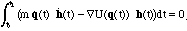and if we integrate by parts, it is: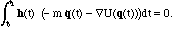(The boundary terms vanish because of the conditions h(t0) = h(t1) = 0, which served to fix the beginning and end of the trajectory.) If, now, we let the three components of h range over a complete set such as sin(n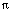t/L), with L = t1-t0, we see that the only possibility is for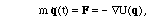which is Newton's law.

The big advantage of Lagrangian mechanics is that it allows us relatively easily to find the equations of motion of an extended body, such as a string. Suppose now that we have a taut string along the x-axis between positions a and b, but displaced laterally by an amount u(t,x). If the density is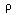, then the kinetic energy of a small bit of string at position x is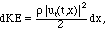so the total kinetic energy is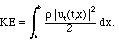It is plausible that the microscopic force transmitted by the string to a point x is proportional to the amount by which the string is stretched at x, i.e., it depends on the arc length element ds at x. As we know,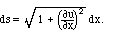Thus we may assume that the differential potential energy depends on u only through ux. The total potential energy would be of the form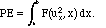The Lagrangian action integral will be the integral of KE - PE with respect to time. Notice that it is u and not x which corresponds to the q used above; the "trajectory" of the string is specified by the function u(t,x), and a variation would entail replacing u(t,x) with
u(t,x) +h(t,x),
where h(t0,x) = h(t1,x) = 0. If the ends of the string are fixed, we would also have

h(t,a) = h(t,b) = 0. The action is a double integral,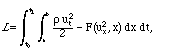and if we use Taylor's theorem to keep only the first-order term inLagrange's condition reads: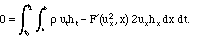This general formula may be useful in deriving realistic wave equations for non-homogeneous strings, but let us simplify at this stage by assuming thatand T := 2 F' are constants. If we integrate the first integrand by parts in the t variable and the second by parts in the x variable, we now find that: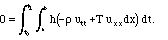If h is arbitrary enough to run through a complete set, we must conclude that

utt = c2 uxx,                                                             (WE)
with c2 = T/a positive constant with dimensions of velocity2.

Judging from guitar strings, for which a 1 meter taut string gives a musical note in the mid range of the musical scale, typical values of c for thin metal strings are on the order of 1000 m./sec. The wave equation (WE) also describes one-dimensional acoustic waves (c ~ 344 m/sec. in air at room temperature or 330 m./sec. at 0 C) and light waves (c ~ 300,000,000 m./sec.), although the physical derivation in these cases is very different.